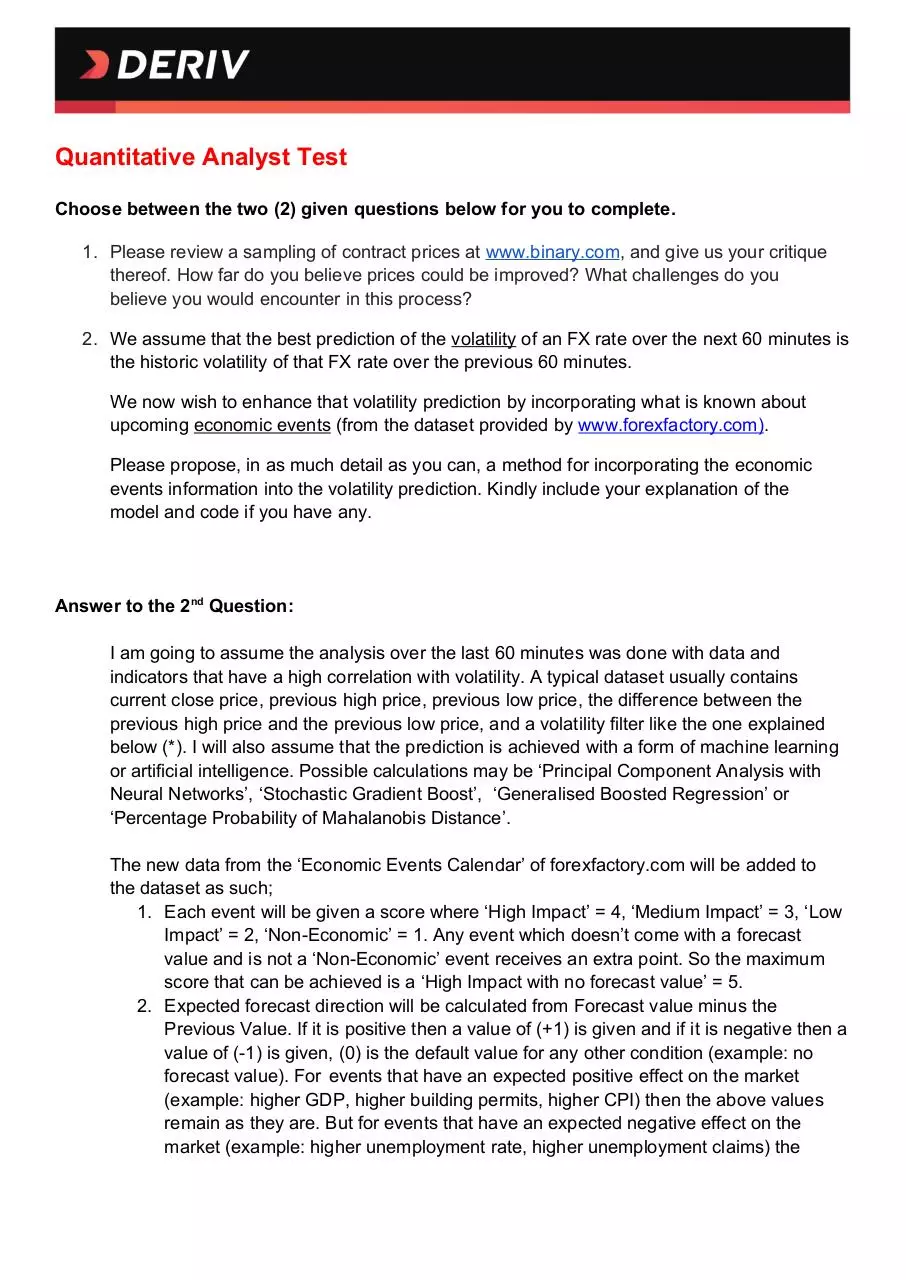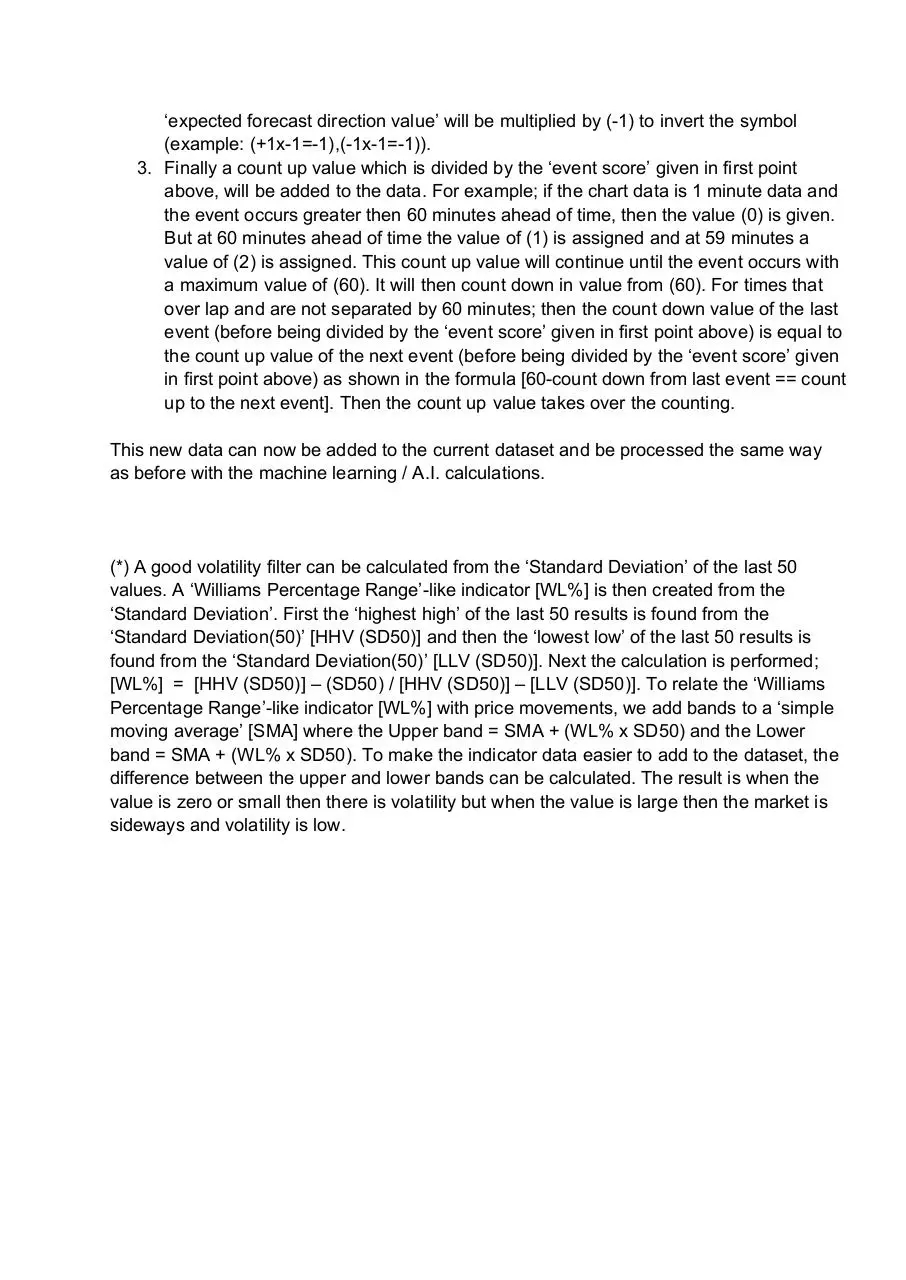# Deriv Quantitative Analyst Answer .pdf

### File information

This PDF 1.4 document has been generated by Online2PDF.com, and has been sent on pdf-archive.com on 29/06/2020 at 03:51, from IP address 202.90.x.x. The current document download page has been viewed 97 times.
File size: 70.78 KB (2 pages).
Privacy: public file

### Document preview

Quantitative Analyst Test
Choose between the two (2) given questions below for you to complete.
1. Please review a sampling of contract prices at www.binary.com, and give us your critique
thereof. How far do you believe prices could be improved? What challenges do you
believe you would encounter in this process?
2. We assume that the best prediction of the volatility of an FX rate over the next 60 minutes is
the historic volatility of that FX rate over the previous 60 minutes.
We now wish to enhance that volatility prediction by incorporating what is known about
upcoming economic events (from the dataset provided by www.forexfactory.com).
Please propose, in as much detail as you can, a method for incorporating the economic
events information into the volatility prediction. Kindly include your explanation of the
model and code if you have any.

I am going to assume the analysis over the last 60 minutes was done with data and
indicators that have a high correlation with volatility. A typical dataset usually contains
current close price, previous high price, previous low price, the difference between the
previous high price and the previous low price, and a volatility filter like the one explained
below (*). I will also assume that the prediction is achieved with a form of machine learning
or artificial intelligence. Possible calculations may be ‘Principal Component Analysis with
Neural Networks’, ‘Stochastic Gradient Boost’, ‘Generalised Boosted Regression’ or
‘Percentage Probability of Mahalanobis Distance’.
The new data from the ‘Economic Events Calendar’ of forexfactory.com will be added to
the dataset as such;
1. Each event will be given a score where ‘High Impact’ = 4, ‘Medium Impact’ = 3, ‘Low
Impact’ = 2, ‘Non-Economic’ = 1. Any event which doesn’t come with a forecast
value and is not a ‘Non-Economic’ event receives an extra point. So the maximum
score that can be achieved is a ‘High Impact with no forecast value’ = 5.
2. Expected forecast direction will be calculated from Forecast value minus the
Previous Value. If it is positive then a value of (+1) is given and if it is negative then a
value of (-1) is given, (0) is the default value for any other condition (example: no
forecast value). For events that have an expected positive effect on the market
(example: higher GDP, higher building permits, higher CPI) then the above values
remain as they are. But for events that have an expected negative effect on the
market (example: higher unemployment rate, higher unemployment claims) the

‘expected forecast direction value’ will be multiplied by (-1) to invert the symbol
(example: (+1x-1=-1),(-1x-1=-1)).
3. Finally a count up value which is divided by the ‘event score’ given in first point
above, will be added to the data. For example; if the chart data is 1 minute data and
the event occurs greater then 60 minutes ahead of time, then the value (0) is given.
But at 60 minutes ahead of time the value of (1) is assigned and at 59 minutes a
value of (2) is assigned. This count up value will continue until the event occurs with
a maximum value of (60). It will then count down in value from (60). For times that
over lap and are not separated by 60 minutes; then the count down value of the last
event (before being divided by the ‘event score’ given in first point above) is equal to
the count up value of the next event (before being divided by the ‘event score’ given
in first point above) as shown in the formula [60-count down from last event == count
up to the next event]. Then the count up value takes over the counting.
This new data can now be added to the current dataset and be processed the same way
as before with the machine learning / A.I. calculations.

(*) A good volatility filter can be calculated from the ‘Standard Deviation’ of the last 50
values. A ‘Williams Percentage Range’-like indicator [WL%] is then created from the
‘Standard Deviation’. First the ‘highest high’ of the last 50 results is found from the
‘Standard Deviation(50)’ [HHV (SD50)] and then the ‘lowest low’ of the last 50 results is
found from the ‘Standard Deviation(50)’ [LLV (SD50)]. Next the calculation is performed;
[WL%] = [HHV (SD50)] – (SD50) / [HHV (SD50)] – [LLV (SD50)]. To relate the ‘Williams
Percentage Range’-like indicator [WL%] with price movements, we add bands to a ‘simple
moving average’ [SMA] where the Upper band = SMA + (WL% x SD50) and the Lower
band = SMA + (WL% x SD50). To make the indicator data easier to add to the dataset, the
difference between the upper and lower bands can be calculated. The result is when the
value is zero or small then there is volatility but when the value is large then the market is
sideways and volatility is low.### Share on social networks

#### HTML Code

Copy the following HTML code to share your document on a Website or Blog